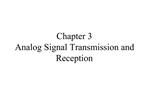DownloadDownload PresentationChapter 3 Analog Signal Transmission and Reception

# Chapter 3 Analog Signal Transmission and Reception

Download Presentation## Chapter 3 Analog Signal Transmission and Reception

- - - - - - - - - - - - - - - - - - - - - - - - - - - E N D - - - - - - - - - - - - - - - - - - - - - - - - - - -
##### Presentation Transcript

1. Chapter 3 Analog Signal Transmission and Reception

3. 3.1 INTRODUCTION TO MODULATION Denote m(t) as the analog signal to be transmitted. The signal m(t) is assumed to be a lowpass signal of bandwidth W and is a power-type signal with The message signal m(t) is transmitted through the communication channel by putting it on a carrier signal of the form

5. 3.2 AMPLITUDE MODULATION (AM) The message signal m(t) is impressed on the amplitude of the carrier signal c(t). Types of amplitude modulation Double-sideband, suppressed carrier AM (DSB-SC AM) Conventional double-sideband AM Single-sideband AM (SSB AM) Vestigial-sideband AM (VSB AM)

6. 3.2.1 Double-Sideband Suppressed Carrier AM A double-sideband, suppressed carrier (DSB-SC) AM signal is obtained by multiplying the message signal m(t) with the carrier signal c(t). Amplitude modulated signal The spectrum of the modulated signal can be obtained by taking the Fourier transform of u(t).

9. The magnitude of the spectrum of the message signal m(t) has been translated or shifted in frequency by an amount The phase of the message signal has been translated in frequency and offset by the carrier phase The bandwidth of the AM signal is 2W, where W is the bandwidth of m(t). The upper (lower) sideband of U(f) contains all the frequency content of the message signal M(f). u(t) does not contain carrier components - u(t) is called a suppressed-carrier signal (DSB-SC AM signal)

55. Frequency Division Multiplexing The message signals are separated in frequency; each signal modulates a separated carrier. The lowpass filters at the transmitter ensures that the bandwidth of the message signals is limited to W Hz. At the receiver, the signals are usually separated by passing through a parallel bank of bandpass filters. The output of each bandpass filter is demodulated.

59. AM-radio Broadcasting Commercial AM-radio broadcasting utilizes the frequency band 535-1605 kHz for the transmission of voice and music. Carrier spacing is 10 kHz. The baseband message signal m(t) is limited to 5 kHz. Conventional AM is used from an economic standpoint. The major objective is to reduce the cost of implementing the receiver.

83. A nonlinear device followed by a bandpass filter tuned to the desired center frequency can be used as frequency multiplier. For example, assume a nonlinear device has the function The output signal will be The frequency is multiplied by a factor of 2.